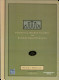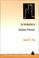### Stochastic Processes

#### Course Description

Conditional and marginal distributions of random variables. Functions of random variables and vectors. Modeling of binomial, Poisson, exponential and normal random variables. Markov chains. Stochastic processes. Markov processes. Poisson and similar processes. Gaussian processes. Kolmogoros?s equations. Spectral analysis of random processes. Processes in linear systems, filtration, estimation and prediction.

#### General Competencies

Learning advanced calculus of stochastic variables and vectors. Acceptance of concepts of stochastic processes

#### Learning Outcomes

1. recognize the type and basic characteristic of the processes in the real environment
2. use the technique of Markov chains in simple modeling
3. recognize stochastic independence and learn to measure dependence of various processes
4. calculate the main parameters of the processes with known distribution type
5. analyze advanced models of stochastic experiments
6. apply stochastic technique in modeling of real linear systems

#### Forms of Teaching

Lectures

Lectures are organized through two cycles. The first cycle consists of 7 weeks of classes and mid-term exam, a second cycle of 6 weeks of classes and final exam. Classes are conducted through a total of 15 weeks with a weekly load of 3 hours.

Exams

Mid-term exam in the 8th week of classes and final exam in the 15th week of classes.

Consultations

Consultations are held one hour weekly according to arrangement with students.

Seminars

Student can choose the topic of an essay and write it during the term.

Continuous Assessment Exam
Quizzes 0 % 20 % 0 % 20 %
Mid Term Exam: Written 0 % 40 % 0 %
Final Exam: Written 0 % 40 %
Exam: Written 0 % 80 %

#### Week by Week Schedule

1. Random variables and vectors. Conditional and marginal distributions. Independence. Conditional expectation. Charecteristic function.
2. Basic distributions. Binomial, Poisson, exponential and normal distribution.
3. Generating function. Properties and applications. Polinomial schema.
4. Random walk. Recursive equations. Two player game. Generalized random walk.
5. Recurrent events. Markov chains. Matrices of transition probabilities.
6. Stationary probabilities. Classification of states of Markov chains.
7. Stochastic processes. Finite-dimensional distributions. Classification of processes. Independence. Correlation functions.
8. Exam.
9. Poisson process. Construction of Poisson process. Sum and decomposition of Poissonovih processes.
10. Homogenous Markov processes. Kolmogorov’s equations. Birth and death process.
11. Brownian motion. Kolmogorov’s equations for diffusion processes.
12. Spectral density and correlation function. White noise. Ergodicity.
13. Linear systems. Transformation of processes. Connection to autocorrelation and cross-correlation functions.
14. Best estimation criteria. Wiener filter. Prediction of processes.
15. Exam.

#### Study Programmes

Computer Engineering (profile)
Mathematics and Science (1. semester)
Computer Science (profile)
Mathematics and Science (1. semester)
Control Engineering and Automation (profile)
Mathematics and Science (1. semester)
Electrical Engineering Systems and Technologies (profile)
Mathematics and Science (1. semester)
Electrical Power Engineering (profile)
Mathematics and Science (1. semester)
Electronic and Computer Engineering (profile)
Mathematics and Science (1. semester)
Electronics (profile)
Mathematics and Science (1. semester)
Information Processing (profile)
Mathematics and Science (1. semester)
Software Engineering and Information Systems (profile)
Mathematics and Science (1. semester)
Telecommunication and Informatics (profile)
Mathematics and Science (1. semester)
Wireless Technologies (profile)
Mathematics and Science (1. semester)

#### Literature

N. Elezović (2010.), Stohastički procesi, FER, interna skripta
J. Medhi (1982.), Stochastic Processes, WELP. Z. Peebles (1987.), Probability, random variables and random signal principles, McGraw-HillE. P. C. Kao (1997.), An Introduction to Stochastic Processes, Wadsworth Publishing Company

#### General

ID 34567
Winter semester
4 ECTS
L0 English Level
L1 e-Learning
45 Lectures
0 Exercises
0 Laboratory exercises
0 Project laboratory